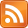Apparently this is a thing. Consider the function $f(x) = \frac{1}{1-x}$. Maybe you know that you can express $f(x)$ as an infinite polynomial:

$$\frac{1}{1 - x} = 1 + x + x^2 + x^3 + x^4 + ...$$

I find this difficult to believe, despite being already familiar with it. Here's a rather standoffish proof: just multiply the right-hand side by $(1 - x)$ and see what you get.

$$\begin{matrix} (1 - x)(1 + x + x^2 + ...) & = & (1 + x + x^2 + ...) - x(1 + x + x^2 + ...)\\ & = & 1 + \color{red}{x} + \color{green}{x^2} + \color{blue}{x^3} + ... - \color{red}{x} - \color{green}{x^2} - \color{blue}{x^3} - ...\\ & = & 1\\ \end{matrix}$$

Okay. We can rescue our intuition a bit by noting that the equality only makes sense when the series converges, i.e. when $|x| < 1$. For example, when $x = 0.5$, we have $$1/(1 - 0.5) = 2 = 1 + 0.5 + 0.5^2 + 0.5^3 + ...$$

This is just a geometric series. It all is, if you think of $x$ as the common ratio between terms. It's also a Taylor series, since we can get it by differentiating $\frac{1}{1 - x}$ a bunch of times. You can also get it by doing polynomial long division -- just like regular division, except you replace $10$ by $x$.

  ____ 1 - x | 1  Consider the first term only. One goes into one one time, so write that at the top.  1 ____ 1 - x | 1  Now multiply $(1 - x)$ by $1$ and write it beneath.  1 ____ 1 - x | 1 - 1 + x  Subtract.  1 ____ 1 - x | 1 - 1 + x ________ 0 + x  How many times does one go into $x$?  1 + x ____ 1 - x | 1 - 1 + x ________ 0 + x x - x^2  Now multiply $(1 - x)$ by $x$ and write it beneath.  1 + x ____ 1 - x | 1 - 1 + x ________ 0 + x - x + x^2 _________ 0 + x^2  Subtract again. We'll put $x^2$ up top, and then do this forever.

But I pulled a bit of a fast one there. Why did I write the divisor as 1 - x instead of -x + 1? We usually put the larger term first (we certainly do in decimal).

It actually changes the answer when you do that. Try dividing $1$ by $-x + 1$ for yourself. (The first thing you have to do is decide how many times $-x$ goes into $1$: that would be $-x^{-1}$ times, which is your first term, which means the answer definitely isn't the same). The answer you get is:

$$\frac{1}{-x + 1} = -x^{-1} - x^{-2} - x^{-3} - ...$$

(Source). But the world hasn't gone mad, $1 - x$ and $-x + 1$ are still equivalent algebraically, so we must have equality to $\frac{1}{1 - x}$ as well. And indeed we do:

$$(1-x)(-x^{-1} - x^{-2} - x^{-3} - ...)$$ $$(-x^{-1} - x^{-2} - x^{-3} - ...) - (-1 - x^{-1} - x^{-2} - x^{-3} - ...)$$ $$(-\color{red}{x^{-1}} - \color{green}{x^{-2}} - \color{blue}{x^{-3}} - ...) + 1 + \color{red}{x^{-1}} + \color{green}{x^{-2}} + \color{blue}{x^{-3}} + ...$$ $$1$$

Until today, I implicitly believed that "Taylor series" and "series expansion" were the same thing. But I'm here to tell you that every Taylor series, converging when $x$ is small, has an evil twin, a mystical equivalent, a hippie cousin: the Laurent series, which converges when $x$ is large. This is the Laurent series for $\frac{1}{1 - x}$.

You can see that, because of all the $x$'s in the denominators, it won't converge unless $|x| > 1$. So it's a perfect complement to the Taylor series, which occupies the $|x| < 1$ space. Both are infinite polynomials that represent the function in some sense.1

Questions: Could there be more types of series expansions? Wolfram Alpha refers to Laurent series as "centered around $\infty$" (vs. Taylor series centered around $0$) -- what does that mean? The forbidding Wikipedia page makes it clear that Laurent series have something to do with $\mathbb{C}$, but what's the relation?

Another Question: Here we've taken a divison problem that ordinarily gives an infinite but convergent answer, and "flipped it around" to get an answer that doesn't converge. Is there anything in doing this for a decmial long division problem, i.e. we keep pulling down larger and larger powers of 10?

 To me, it even makes a loose kind of sense which ordering gives you which series. Suppose you're always putting the most significant terms on the left, to mimick positional number systems. Then, writing $1 + x$ means that $|x| < 1$, and this gives you the Taylor series which is only true for $|x| < 1$.

[Edited 10/31/2019 to remove an incorrect statement.]